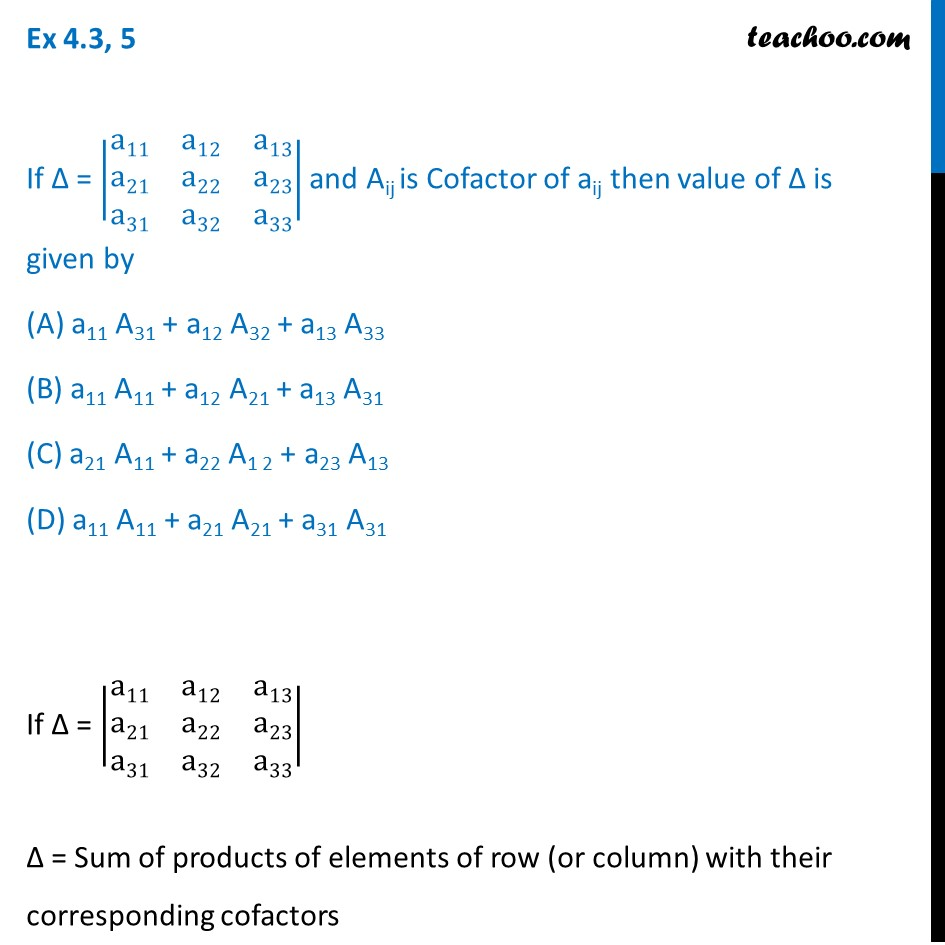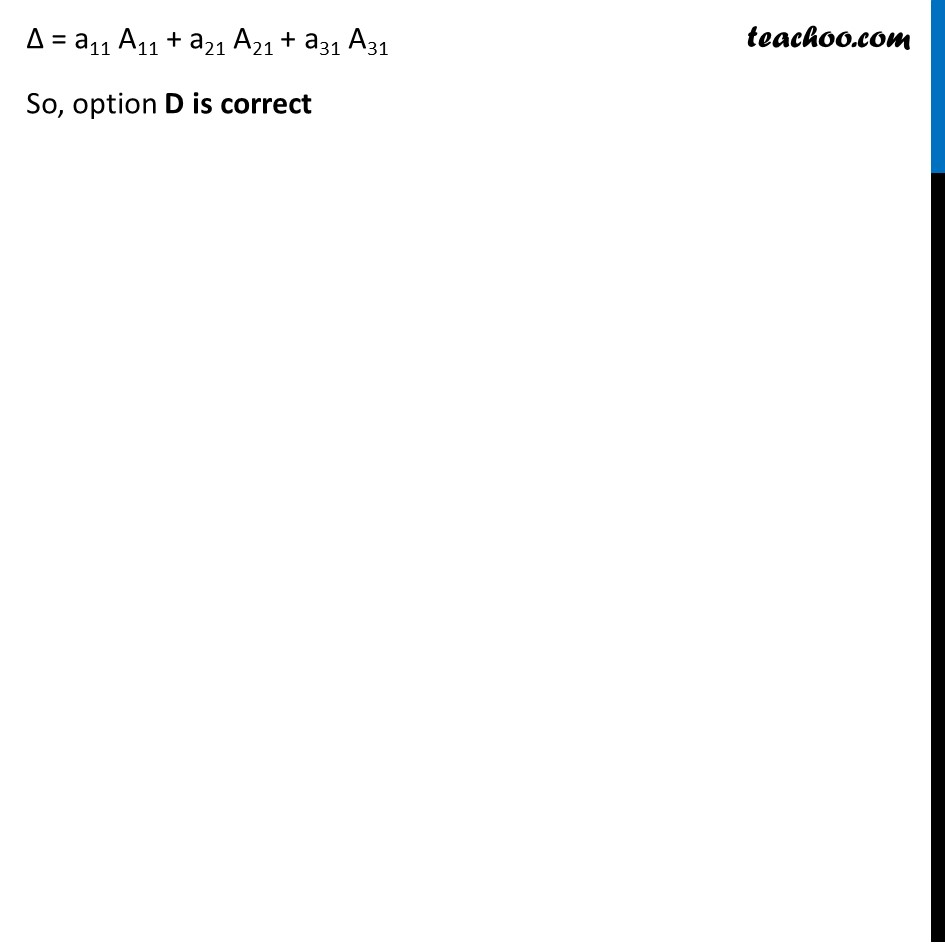Ex 4.3

Chapter 4 Class 12 Determinants
Serial order wiseLearn in your speed, with individual attention - Teachoo Maths 1-on-1 Class

### Transcript

Ex 4.3, 5 If ∆ = |■8(a11&a12&a13@a21&a22&a23@a31&a32&a33)| and Aij is Cofactor of aij then value of ∆ is given by (A) a11 A31 + a12 A32 + a13 A33 (B) a11 A11 + a12 A21 + a13 A31 (C) a21 A11 + a22 A1 2 + a23 A13 (D) a11 A11 + a21 A21 + a31 A31 If ∆ = |■8(a11&a12&a13@a21&a22&a23@a31&a32&a33)| ∆ = Sum of products of elements of row (or column) with their corresponding cofactors ∆ = a11 A11 + a21 A21 + a31 A31 So, option D is correct